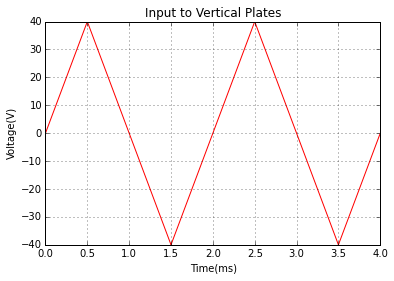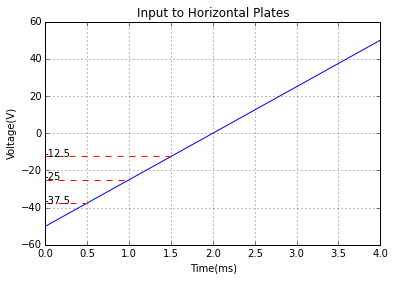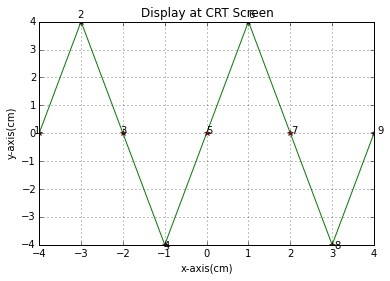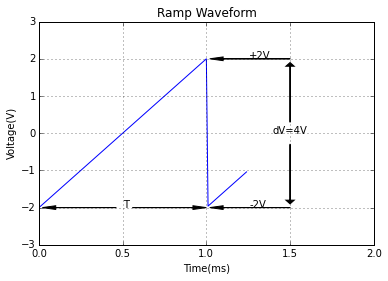# CHAPTER 9: CATHODE-RAY OSCILLOSCOPES¶

## Example 9-1, Page Number: 238¶

In :
import numpy as np
import matplotlib.pyplot as plt
%matplotlib inline

#To Obtain plot seen on CRT screen when triangular wave of peak voltage 40V and frequency 500 Hz
#Time Base is sawtooth of 250Hz
#As the triangular wave has increasing and decreasing parts, it is plotted piecewise
#Time scale is divided into 5 regions

t=np.arange(0.0,4.0,.001)              #Total time scale

#Time Scale division
t1=np.arange(0.001,0.5,0.001)
t2=np.arange(0.5,1.5,0.001)
t3=np.arange(1.5,2.5,0.001)
t4=np.arange(2.5,3.5,0.001)
t5=np.arange(3.5,4.0,.001)

#To plot vertical plate input
plt.plot(t1,80*t1,'r')               #Plot the graph piecewise
plt.plot(t2,-80*t2+80,'r')
plt.plot(t3,80*t3-160,'r')
plt.plot(t4,-80*t4+240,'r')
plt.plot(t5,80*t5-320,'r')
plt.grid(True)
plt.xlabel('Time(ms)')
plt.ylabel('Voltage(V)')
plt.title('Input to Vertical Plates')
plt.show()

#To plot horizontal plate input
plt.plot(t,25*t-50)
t11=np.arange(0.001,0.5,0.001)
t12=np.arange(0.001,1,0.001)
t13=np.arange(0.001,1.5,.001)
plt.plot(t11,-37.5*t11/t11,'--r')
plt.plot(t12,-25*t12/t12,'--r')
plt.plot(t13,-12.5*t13/t13,'--r')
plt.annotate("-37.5",(0,-37.5))
plt.annotate("-25",(0,-25))
plt.annotate("-12.5",(0,-12.5))
plt.grid(True)
plt.xlabel('Time(ms)')
plt.ylabel('Voltage(V)')
plt.title('Input to Horizontal Plates')
plt.show()

#CRT screen plot, Horizontal deflection sensitivity=0.08cm/V and Vertical deflection sensitivity is 0.1cm/V

fig = plt.figure()

#Plotted piecewise
#The deflection senstivities are multiplied to convert voltage to cm
plt.plot(0.08*(25*t1-50),0.1*(80*t1),'g')
plt.plot(0.08*(25*t2-50),0.1*(-80*t2+80),'g')
plt.plot(0.08*(25*t3-50),0.1*(80*t3-160),'g')
plt.plot(0.08*(25*t4-50),0.1*(-80*t4+240),'g')
plt.plot(0.08*(25*t5-50),0.1*(80*t5-320),'g')
A=[-4,-3,-2,-1,0,1,2,3,4]
B=[0,4,0,-4,0,4,0,-4,0]
plt.plot(A,B,'r*')
i=1
for xy in zip(A, B):
ax.annotate('%d' % i, xy=xy, textcoords='offset points')
i=i+1
ax.xaxis.set_ticks(A)
ax.grid(True)
plt.xlabel('x-axis(cm)')
plt.ylabel('y-axis(cm)')
plt.title('Display at CRT Screen')
plt.show()## Example 9-2, Page Number: 243¶

In :
import numpy as np
import matplotlib.pyplot as plt
%matplotlib inline
#Variable Declaration

R3=4.2*10**3      #Collector resistance
C1=0.25*10**-6    #Capacitance connected to emitter of transistor
Vb1=4.9           #Voltage across R1 as shown in diagram
Vt=2              #Modulus of upper and lower trigger levels
Vbe=0.7           #Base-Emitter Voltage Drop of transistor

#Calculation
dV=2*Vt           #Peak to Peak of ramp signal

Ic1=(Vb1-Vbe)/R3  #Collector Current
T=dV*C1/Ic1       #Ramp time period
print "Time period=",round(T*1000),"ms"
#Plot of ramp signal

t=np.arange(0,1.25,0.01)
x=np.zeros(125)

for i in range (0,125):
if(i<=100):
x[i]=4*i*0.01-2
else:
x[i]=4*i*0.01-6

plt.plot(t,x)
plt.ylim(-3,3)
plt.xlim(0,2)
plt.annotate("dV=4V",(1.4,0))
plt.annotate("T",(0.5,-2))
plt.annotate("+2V",(1.26,2))
plt.annotate("-2V",(1.26,-2))
plt.grid(True)
plt.xlabel('Time(ms)')
plt.ylabel('Voltage(V)')
plt.title('Ramp Waveform')

Time period= 1.0 ms

Out:
<matplotlib.text.Text at 0x7e64710>## Example 9-3, Page Number 256¶

In :
import math

#Variable Declarataion
voltage_per_div=200*10**-3       #Voltage sensitivity(V/div)
time_per_div=0.1*10**-3          #Time Scale sensitivity (s/div)
Dva=6                            #Vertical distance betweeen peaks of A(div)
Dha=6                            #Horizontal distance between peaks of A(div)
Dvb=2.4                          #Vertical distance between peaks of B(div)
Dhb=6                            #Horizontal distance between peaks of B(div)
phase_difference=1               #Phase difference(div)

#Calculation
Vapp=Dva*voltage_per_div          #Peak to Peak voltage of A
Ta=Dha*time_per_div               #Time period of A
fa=1/Ta                           #Frequency of A

Vbpp=Dvb*voltage_per_div
Tb=Dhb*time_per_div
fb=1/Tb

phase_difference_angle=360*phase_difference/6   #360 degrees corresponds to 6 divisions on time scale.
#Thus phase angle corresponding to 1 division is found
#Results
print "Waveform A"
print "Peak to Peak Voltage=",round(Vapp),"V"
print "Frequency=",int(fa)+4,"Hz"
print
print "Waveform B"
print "Peak to Peak Voltage=",round(Vbpp),"V"
print "Frequency=",int(fb)+4,"Hz"
print
print "Phase difference between A and B is",phase_difference_angle,"degrees"

Waveform A
Peak to Peak Voltage= 1.0 V
Frequency= 1670 Hz

Waveform B
Peak to Peak Voltage= 0.0 V
Frequency= 1670 Hz

Phase difference between A and B is 60 degrees


## Example 9-4, Page Number: 259¶

In :
import math

#Variable Declaration
voltage_per_div=2          #in V/div
time_per_div=5*10**-6      #in s/div
Dv=4                       #Vertical Distance(div)
Dh=5.6                     #Horizontal distance(div)
Dhr=0.5                    #Rise time distance(div)
Dhf=0.6                    #Fall time distance(div)
#Calculation
PA=Dv*voltage_per_div      #Pulse Amplitude
T=Dh*time_per_div          #Time Period
f=1/T                      #Frequency
tr=Dhr*time_per_div        #Rise Time
tf=Dhf*time_per_div        #Fall Time

#Results

print "Pulse Amplitude=",int(PA),"V"
print "Frequency=",round(f/1000,1),"kHz"
print "Rise Time=",round(tr*10**6,1),"micro second"
print "Fall Time=",round(tf*10**6),"micro second"

Pulse Amplitude= 8 V
Frequency= 35.7 kHz
Rise Time= 2.5 micro second
Fall Time= 3.0 micro second


## Example 9-5, Page Number: 261¶

In :
import math

#Variable Declaration
Ri=10*10**6       #in ohm

#Calculation
T=Ri*Cc           #Time Constant
PW=T/10           #Pulse Width

#Results

print "Time Constant=",int(T),"s"
print "Longest Pulse Width=",int(PW*1000),"ms"

Time Constant= 1 s
Longest Pulse Width= 100 ms


## Example 9-6, Page Number 262¶

In :
import math

#Variable Declaration
Rs=3.3*10**3
Ci=15*10**-12

#Calculation
tro=2.2*Rs*Ci    #Time constant imposed by oscilloscope
PWmin=10*tro     #Minimum pulse width

#Results

print "tro=",round(tro*10**9),"ns"
print "PWmin=",round(PWmin*10**6,2),"micro second"

tro= 109.0 ns
PWmin= 1.09 micro second


## Example 9-7, Page Number: 262¶

In :
import math

#Variable Declaration
tri1=109*10**-9                #Input rise time for case a(second)
tri2=327*10**-9                #Input rise time for case b(second)
R=3.3*10**3                    #in ohm

#Calculation
tro=2.2*R*C                    #Time constant due to oscilloscope
#When tri=109ns

trd1=math.sqrt(tri1**2+tro**2) #Displayed rise time for case a

#When tri=327ns
trd2=math.sqrt(tri2**2+tro**2) #Displayed rise time for case b

#Results

print "When input pulse rise time is 109ns, trd=",round(trd1*10**9),"ns"
print "When input pulse rise time is 327ns, trd=",round(trd2*10**9),"ns"

When input pulse rise time is 109ns, trd= 154.0 ns
When input pulse rise time is 327ns, trd= 345.0 ns


## Example 9-8, Page Number : 264¶

In :
import math

#Variable Declaration
Vs=1                  #Input signal voltage(V)
Rs=600.0                #Source resistance(ohm)
Ri=1*10**6            #Input Impedance(ohm)
f=100                 #Signal frequency(Hz)

#Calculation
Ct=Ci+Ccc             #Total capacitance:Addition of parallel capaciatances
#At 100 Hz,
Xc=1/2*pi*f*Ct        #Capacitvie reactance of total capacitance
Vi=Vs*Ri/(Rs+Ri)      #Voltage Divider rule is used as Xc>>Rs and Ri

#When Vi=Vs-3dB
f1=1/(2*pi*Ct*Rs)       #When vi is 3db less than Vs, Xc=Rs

#Results

print "When signal frequence is 100Hz, oscilloscope terminal voltage (Vi)=",round(Vi,4),"V"
print "When Vi is 3dB less than Vs, f=",round(f1*10**-6,2),"MHz"

When signal frequence is 100Hz, oscilloscope terminal voltage (Vi)= 0.9994 V
When Vi is 3dB less than Vs, f= 2.04 MHz


## Example 9-9, Page Number: 267¶

In :
import math

#Variable Declaration

#As C1 is required to compensate 10:1 probe
R1=9*10**6
Ri=1*10**6

#Calculation
Ct=1/(1/C1+1/C2)    #Probe capacitance(farad). Equivalent of series capacitance

#Results

print "The value of C1 required to compensate a 10:1 probe is",round(C1*10**12,1),"pF"
print "The input capacitance seen from the source is",round(Ct*10**12),"pF"

The value of C1 required to compensate a 10:1 probe is 14.4 pF
The input capacitance seen from the source is 13.0 pF


## Example 9-10, Page Number 268¶

In :
import math

#Variable Declaration
Rs=600            #Source resistance(ohm)

#For 3 dB reduction, Xc=Rs

f=1/(2*pi*Rs*C)   #Frequency for 3dB reduction(Hz)

print "The signal frequency at which the probe casues a 3dB reduction in the signal is,",round(f*10**-6,1),"MHz"

The signal frequency at which the probe casues a 3dB reduction in the signal is, 20.4 MHz


## Example 9-11, Page Number: 269¶

In :
import math

#Variable Declaration
Rs=600                 #Source resistance (ohm)

#Calcualtion
f=1/(2*pi*C*Rs)        #Frequency at which Xc=Rs(Hz)

#Result
print "The frequency for 3dB reduction is,",round(f*10**-6,1),"MHz"


The frequency for 3dB reduction is, 75.8 MHz


## Example 9-12, Page Number: 278¶

In :
import math

#Variable Declaration
f=50.0*10**6                #Frequency of waveform(Hz)
expansion_factor=5      #Time base magnifier expansion factor

#Calculation
T=1/f                     #Time period

#For one cycle occupying four horizontal divisions,
minimum_time_per_div=T/4
#Using the five-times magnifier to give 5ns/div
minimum_time_per_div_setting=minimum_time_per_div*expansion_factor

#Result
print "Minimum time/division senstivity=",minimum_time_per_div_setting*10**9,"ns/div"

Minimum time/division senstivity= 25.0 ns/div


## Example 9-13, Page Number: 279¶

In :
import math

#Variable Declaration
tri=21*10**-9           #Input rise time(s)
fh1=20*10**6            #Upper cut-off frequency for case a(Hz)
fh2=50*10**6            #Upper cut-off frequency for case b(Hz)

#Calculation

#For fh=20 MHz
tro1=0.35/fh1           #Oscilloscope rise time for case a(s)

trd1=math.sqrt(tri**2+tro1**2)  #Display rise time

#For fh=50 MHz
tro2=0.35/fh2                   #Oscilloscope rise time
trd2=math.sqrt(tri**2+tro2**2)  #Display rise time

#Results

print "When fh=20 MHz,"
print "tro=",round(tro1*10**9,1),"ns"
print "trd=",round(trd1*10**9),"ns"
print
print "When fh=50 MHz,"
print "tro=",round(tro2*10**9,1),"ns"
print "trd=",round(trd2*10**9),"ns"

When fh=20 MHz,
tro= 17.5 ns
trd= 27.0 ns

When fh=50 MHz,
tro= 7.0 ns
trd= 22.0 ns